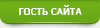» » 8 common pitfalls in JavaScript

### 8 common pitfalls in JavaScript

Author: bamboo06 on 1-04-2020, 19:02, views: 906

1
javascript uses alphanumeric to sort by default. Therefore, the result of [1,2,5,10] .sort () is [1, 10, 2, 5]. If you want to sort correctly, you should do this: [1,2,5,10] .sort ((a, b) => a-b)

1. Have you tried sorting the array elements?
javascript uses alphanumeric to sort by default. So the result of [1,2,5,10] .sort () is [1, 10, 2, 5].
If you want to sort correctly, you should do this: [1,2,5,10] .sort ((a, b) => a-b)

2. new Date () is very easy to use
The methods for using new Date () are:
Does not receive any parameters: returns the current time;
Takes a parameter x: returns the value of January 1, 1970 + x milliseconds.
new Date (1, 1, 1) returns February 1, 1901.
However, new Date (2016, 1, 1) does not add 2016 to the 1900, but only indicates 2016.

3. Is the replacement function not really replaced?
``````let s = "bob" const replaced = s.replace ('b', 'l')
replaced === "lob" // will only replace the first bs === "bob" // and the value of s will not change``````

If you want to replace all b, use regular:
``"bob".replace(/b/g, 'l') === 'lol'``

4. Be careful with comparison operations
``````// these can be 'abc' === 'abc' // true1 === 1 // true // but these cannot
[1,2,3] === [1,2,3] // false {a: 1} === {a: 1} // false {} === {} // false``````

Because [1,2,3] and [1,2,3] are two different arrays, but they happen to have the same elements. Therefore, we cannot simply judge by ===.

5. Arrays are not primitive types
``typeof {} === 'object' // truetypeof 'a' === 'string' // truetypeof 1 === number // true // but ... typeof [] === 'object' // true``

To determine if a variable var is an array, you need to use Array.isArray (var).

6. Closures
This is a classic javascript interview question:
``````const Greeters = []for (var i = 0 ; i < 10 ; i++) {
Greeters.push(function () { return console.log(i) })
}
Greeters() // 10Greeters() // 10Greeters() // 10``````

Although it is expected to output 0,1,2, ..., it is not. Any idea how to debug it? There are two ways:
a. Use let instead of var.
b. Use the bind function.
``Greeters.push(console.log.bind(null, i))``

Of course, there are many solutions. These two are my favorite!

What will the following code output?
``````class Foo {  constructor (name) {    this.name = name
}
greet () {
console.log('hello, this is ', this.name)
}
someThingAsync () {    return Promise.resolve()
}
asyncGreet () {    this.someThingAsync()
.then(this.greet)
}
}
new Foo('dog').asyncGreet()``````

If you say that the program crashes and gives an error: Cannot read property 'name' of undefined. Because greet is not executed in the right environment. Of course, there are many ways to solve this bug!
I like to use the bind function to solve the problem:
``````asyncGreet () {  this.someThingAsync()
.then(this.greet.bind(this))
}``````

This will ensure that the greet will be called by the Foo instance, not the this of the local function.
If you want the greet to never be bound to the wrong scope, you can use bind in the constructor.
``````class Foo {  constructor (name) {    this.name = name    this.greet = this.greet.bind(this)
}
}``````

You can also use the arrow function (=>) to prevent the scope from being modified. Note: You can refer to another blog by Fundebug. Beginners of javascript must see "arrow functions".
``````asyncGreet () {  this.someThingAsync()
.then(() => {    this.greet()
})
}``````

8. Math.min () is larger than Math.max ()
``Math.min() < Math.max() // false``

Because Math.min () returns Infinity, and Math.max () returns -Infinity.

Category: Javascript

Dear visitor, you are browsing our website as Guest.
We strongly recommend you to register and login to view hidden contents.
<• 0 Articles
8 April 2020 00:26